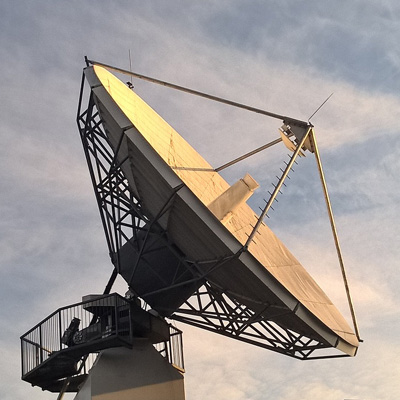This course uses mathematical functions to solve real-world problems. The course discusses trigonometric identities and the law of sines and cosines. Other primary topics include vectors, polar functions, parametric equations, conic sections, matrices and solving systems of linear equations, and combinatorics and probability. This is the second course in a two-part precalculus series (PRECALC 041 and PRECALC 043).

Note